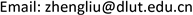MOSModeling and Simulation2324-8696Scientific Research Publishing10.12677/MOS.2021.101008MOS-40298MOS20210100000_29192041.pdf信息通讯 工程技术 一种36 kVA高频变压器的建模仿真与优化 Modeling and Optimization of a 36 kVA High-Frequency Transformer 振堂21红叶21志强313*中国石化大连石油化工研究院，辽宁 大连大连理工大学电气工程学院，辽宁 大连null0302202110017082© Copyright 2014 by authors and Scientific Research Publishing Inc. 2014This work is licensed under the Creative Commons Attribution International License (CC BY). http://creativecommons.org/licenses/by/4.0/

Modeling and Optimization of a 36 kVA High-Frequency Transformer

Zhentang Shi1, Hongye Li1, Zhiqiang Wang2, Zheng Liu2

1Sinopec Dalian Petrochemical Research Institute, Dalian Liaoning

2School of Electrical Engineering, Dalian University of Technology, Dalian LiaoningReceived: Nov. 17th, 2020; accepted: Jan. 30th, 2021; published: Feb. 7th, 2021ABSTRACT

In the fields of DC transmission, photovoltaic power generation and electric locomotive traction, the power electronic technology provides a critical support. Furthermore, the high-frequency transformer is a key component of the power electronic equipment. In recent years, with the application of nanocrystalline materials in manufacture of high-frequency transformers, the performance of transformers has been greatly improved. However, there are still many difficulties in the design of high-frequency transformers with relatively large power in terms of reducing parasitic parameters and increasing power density. This research uses the nanocrystalline as the magnetic core, modeling a 36 kVA transformer with10 kHz and making numerical optimization of total loss and parasitic parameters. Finally, computer simulation technology was used to verify the feasibility and validity of the optimization, and the optimal design parameters were obtained by the comparison.

Keywords:High-Frequency Transformer, Electromagnetic Modeling, Optimization

Copyright © 2021 by author(s) and Hans Publishers Inc.

This work is licensed under the Creative Commons Attribution International License (CC BY 4.0).

http://creativecommons.org/licenses/by/4.0/1. 引言

2. 高频变压器的建模

High frequency transformer parameter

2.1. 高频变压器的数学模型

L σ = L σ 1 + N 1 2 N 2 2 L σ 2 (1)

C s = C 1 + N 2 2 N 1 2 C 2 + ( N 2 N 1 − 1 ) 2 C 12 (2)

2.2. 最大磁通密度B<sub>m</sub>的选择

P c = k c f α B m β V c k f (3)

P m = R a c 1 I 1 2 + R a c 2 I 2 2 = F r 1 N 1 ρ l m 1 S 1 I 1 2 + F r 2 N 2 ρ l m 2 S 2 I 2 2

( 5.35 )

N 1 = U 1 4 B m A c f ,     N 2 = U 2 4 B m A c f (4)

P s = P c + P m (5)

B z β + 1 = F r 1 U 1 ρ l m 1 S 1 I 1 2 + F r 2 U 2 ρ l m 2 S 2 I 2 2 4 A c f α + 1 k m β V c k f (6)

2.3. 建模与理论参数计算

Diameter of nanocrystalline CC core
ABCDEF磁芯截面积Ac窗口面积Wa
40 mm5 mm20 mm50 mm125 mm200 mm20 cm254 cm2

Parameters of nanocrystalline CC core

N 1 = U 1 4 B m A c f = 48   匝 (7)

N 2 = U 2 4 B m A c f = 16   匝 (8)

I 1 = P U 1 = 40   A (9)

I 2 = P U 2 = 120   A (10)

δ = 66.1 f = 66.1 10000 = 0.661   mm (11)

S 1 = I 1 J = 16   mm 2 (12)

S 2 = I 2 J = 48   mm 2 (13)

Winding type and winding parameter

h 1 = h 2 = 104 × 1.15 = 119.6   mm < 120   mm (14)

d = ( 2 + 1.23 × 3 + 0.92 × 2 × 2 + 2 × 4 + 3 ) × 2 = 40.74   mm < 45   mm (15)

F r 1 = 6.08 ,     F r 2 = 1.87 (16)

R d c 1 = l 1 γ S 1 = 16 × ( 196.43 + 216.73 + 237.02 ) 5.8 × 10 7 × 16 × 100 = 0.0112   Ω (17)

R d c 2 = l 2 γ S 2 = 8 × ( 259.23 + 283.36 ) 5.8 × 10 7 × 48 × 100 = 0.0037   Ω (18)

R a c 1 = F r 1 R d c 1 = 0.0681   Ω (19)

R a c 2 = F r 2 R d c 2 = 0.0070   Ω (20)

Theoretical calculation parameters of high frequency transformer with equivalent circuit

Performance of theoretical model of the high frequency transforme

3. 高频变压器的优化与仿真验证

3.1. 高频变压器优化方程

x = ( J ; d j ) ∈ ℝ 2 (21)

min P s ( x )

s .t .   d ( x ) ≤ 45

d 1 ( x ) ≤ 2 δ

d 2 ( x ) ≤ 4 δ

Δ τ ( x ) ≤ 40

η ≥ 0.99

Δ U ( x ) ≤ 0.01

d j ≥ 1.5

J ≤ 5

min L σ ( x )

s .t .   d ( x ) ≤ 45

d 1 ( x ) ≤ 2 δ

d 2 ( x ) ≤ 4 δ

Δ τ ( x ) ≤ 40

η ≥ 0.99

Δ U ( x ) ≤ 0.01

d j ≥ 1.5

J ≤ 5

3.2. 高频变压器优化结果

Optimization result

Performance of the optimized high frequency transforme

3.3. 仿真验证高频变压器优化结果

3.4. 初始高频变压器模型仿真结果

Initial transformer simulation model circuit paramete

Performance of Initial Transformer simulatio

298.270.74%214.8899.39%

3.5. 优化后高频变压器仿真结果

Optimized transformer simulation circuit parameter

Performance of the optimized transformer simulatio

298.760.41%156.7899.56%

3.6. 检验漏感优化效果

Equivalent circuit distribution parameters for high frequency transformer

4. 结论